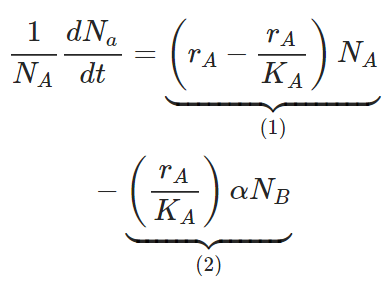# What do each of the two terms labeled as 1 and 2 represent in this equation?

What do each of the two terms labeled as 1 and 2 represent in this equation?a) 1=Effect of species A on growth rate of species B; 2=Effect of species B on growth rate of species A
b) 1=Density-dependent growth rate of species A; 2=Density-dependent growth rate of species B
c) 1=Effect of species A on growth rate of species B; 2=Density-dependent growth rate of species B
d) 1=Density-dependent growth rate of species A; 2=Effect of species B on growth rate of species A